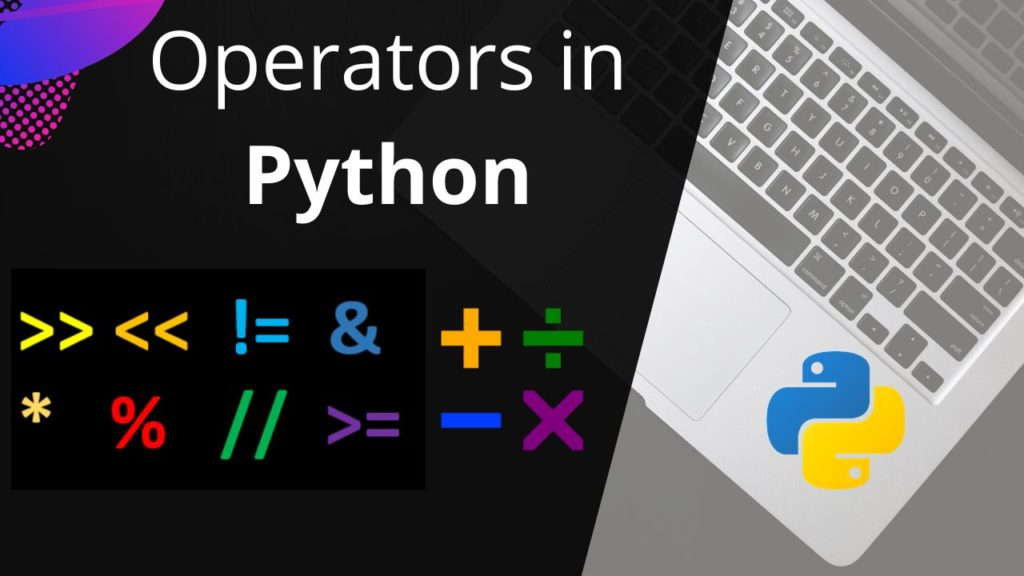# Operators in PythonWhat are operators in Python?

Operators are special symbols in Python that carry out arithmetic or logical computation.

The value that the operator operates on is called the operand.

For example:

``````>>> 5+5
10``````

Here, + is the operator which we use for addition

There are different types of operators which are used in Python

1. Arithmetic operators
2. Assignment operators
3. Relational operators
4. Logical operators
5. Operators Precedence

Arithmetic Operators

Different Arithmetic operators like-

• Subtraction (-) for subtracting second number from first
• Multiplication (*) for multiplying two numbers
• Division (/) for dividing numerator by denominator
• Modulus operator (%) for determining remainder of after an integer division

Assignment Operators

Assignment operator (=) `a = 5` is a simple assignment operator that assigns the value 5 on the right to the variable a on the left.

Relational operators

Different relational operators supported in Python are:

1. Equals (==)
2. Not equals (!=)
3. Greater than (>)
4. Less than (<)
5. Greater or equal to (>=)
6. Less than or equal to (<=)

Logical Operator

Logical operators are the `and``or``not` operators.

Operators Precedence

The combination of values, variables, operators and functions calls is termed as an expression. The Python interpreter can evaluate a valid expression.

For example:

``````>>> 5 - 7
-2``````

Here `5 - 7` is an expression. There can be more than one operator in an expression.

To evaluate these types of expressions there is a rule of precedence in Python. It guides the order in which these operations are carried out.

For example, multiplication has higher precedence than subtraction.

``````
>>> 10 - 4 * 2
2``````

But we can change this order using parentheses `()` as it has higher precedence than multiplication.

``````
>>> (10 - 4) * 2
12``````

The operator precedence in Python is listed in the following table. It is in descending order (upper group has higher precedence than the lower ones).

Operators which have higher precedence appears on the top of the table.